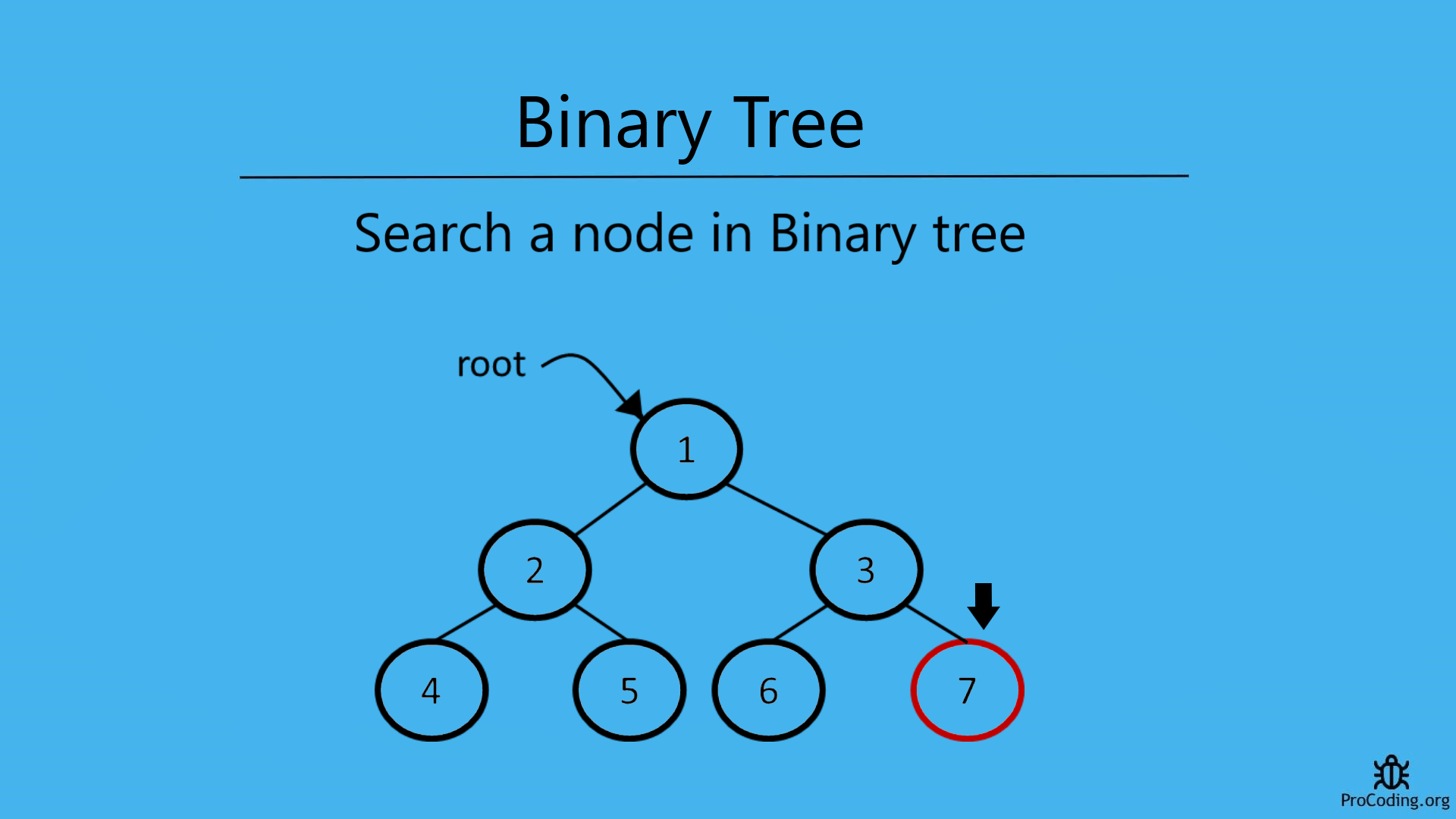# Search a node in Binary tree

Abhishek Kumar Category: Data StructuresTags: datastructures binary-tree

Search a node in a Binary tree using recursion or iterative solution. For example, a binary tree contains the following elements-
1, 2, 3, 4, 5, 6, 7
Here, if 7 is searched then return true but if 9 is searched then return false because it is not available in the binary tree.In a Binary Tree, to search a node we have to traverse each node and check if that node is available in the tree.

We can solve this problem in two ways-

### Recursive solution

For recursive solution we have to check each node one by one recursively as we have done in binary tree recursive traversal and if required node is found then return True otherwise return False.

``````class Node:
def __init__(self, data):
self.left = None
self.data = data
self.right = None

def search_node(root, num):
if root is None:
return False
if root.data == num:
return True
val = search_node(root.left, num)
if val:
return True
return search_node(root.right, num)

root = Node(1)
root.left = Node(2)
root.right = Node(3)
root.left.left = Node(4)
root.left.right = Node(5)
root.right.left = Node(6)
root.right.right = Node(7)
print(search_node(root, 7))``````
``````class Node {
constructor(data) {
this.left = null;
this.data = data;
this.right = null;
}
}

function searchNode(root, num) {
if (!root) return false;
if (root.data === num) return true;
let val = searchNode(root.left, num);
if (val) return true;
return searchNode(root.right, num);
}

const root = new Node(1);
root.left = new Node(2);
root.right = new Node(3);
root.left.left = new Node(4);
root.left.right = new Node(5);
root.right.left = new Node(6);
root.right.right = new Node(7);
console.log(searchNode(root, 7));``````

Output

`True`

### Iterative solution

For iterative solution, we have to traverse each node in level order and if required node is found then return True otherwise return False.

``````import queue

class Node:
def __init__(self, data):
self.left = None
self.data = data
self.right = None

def search_node(root, num):
if root is None:
return False
q = queue.Queue()
q.put(root)
while not q.empty():
node = q.get()
if node.data == num:
return True
if node.left:
q.put(node.left)
if node.right:
q.put(node.right)
return False

root = Node(1)
root.left = Node(2)
root.right = Node(3)
root.left.left = Node(4)
root.left.right = Node(5)
root.right.left = Node(6)
root.right.right = Node(7)
print(search_node(root, 7))``````
``````class Node {
constructor(data) {
this.left = null;
this.data = data;
this.right = null;
}
}

function searchNode(root, num) {
if (!root) return false;

const q = [];
q.push(root);

while (q.length > 0) {
let node = q.shift();
if (node.data === num) return true;
if (node.left) q.push(node.left);
if (node.right) q.push(node.right);
}
return false;
}

const root = new Node(1);
root.left = new Node(2);
root.right = new Node(3);
root.left.left = new Node(4);
root.left.right = new Node(5);
root.right.left = new Node(6);
root.right.right = new Node(7);
console.log(searchNode(root, 7));``````

#### Output

Output

`True`

Time complexity: The time complexity of both of these solution will be O(n) because we are just traversing each element of node one by one.

AUTHOR### Abhishek Kumar

Software engineer | Blogger | Keen learner
Python | Django | JavaScript | React | Next.js | Express.js | C
Passionate about learning and experimenting with new things and open to more opportunities and collaborations.